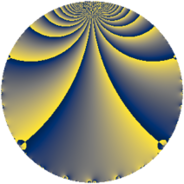Properties

 Label 43.2.eLevel $43$ Weight $2$ Character orbit 43.e Rep. character $\chi_{43}(4,\cdot)$ Character field $\Q(\zeta_{7})$ Dimension $12$ Newform subspaces $2$ Sturm bound $7$ Trace bound $2$

Related objects

Defining parameters

 Level: $$N$$ $$=$$ $$43$$ Weight: $$k$$ $$=$$ $$2$$ Character orbit: $$[\chi]$$ $$=$$ 43.e (of order $$7$$ and degree $$6$$) Character conductor: $$\operatorname{cond}(\chi)$$ $$=$$ $$43$$ Character field: $$\Q(\zeta_{7})$$ Newform subspaces: $$2$$ Sturm bound: $$7$$ Trace bound: $$2$$ Distinguishing $$T_p$$: $$2$$

Dimensions

The following table gives the dimensions of various subspaces of $$M_{2}(43, [\chi])$$.

Total New Old
Modular forms 24 24 0
Cusp forms 12 12 0
Eisenstein series 12 12 0

Trace form

 $$12 q - 2 q^{2} - q^{3} - 2 q^{4} - q^{5} - 2 q^{6} - 16 q^{7} - 6 q^{8} - 7 q^{9} + O(q^{10})$$ $$12 q - 2 q^{2} - q^{3} - 2 q^{4} - q^{5} - 2 q^{6} - 16 q^{7} - 6 q^{8} - 7 q^{9} + 7 q^{10} + 4 q^{11} + 11 q^{12} - 4 q^{13} - 15 q^{14} - 9 q^{15} + 8 q^{16} + 4 q^{17} + 13 q^{18} - 2 q^{19} + 15 q^{20} + 8 q^{21} + 6 q^{22} + 2 q^{23} - 4 q^{24} - 3 q^{25} + 3 q^{26} + 29 q^{27} + 21 q^{28} + 15 q^{29} - 16 q^{30} - 24 q^{31} - 36 q^{32} + 20 q^{33} + 12 q^{34} + 4 q^{35} - 16 q^{36} - 18 q^{37} - 27 q^{38} + 5 q^{39} + 19 q^{40} + 25 q^{41} - 38 q^{42} - 42 q^{43} - 62 q^{44} - 26 q^{45} + 16 q^{46} + 18 q^{47} + 11 q^{48} - 4 q^{49} + 12 q^{50} - 24 q^{51} + 3 q^{52} - 12 q^{53} + 42 q^{54} + 19 q^{55} + 9 q^{56} - 9 q^{57} - 25 q^{58} + 34 q^{59} + 33 q^{60} + 31 q^{61} + 45 q^{62} + 9 q^{63} + 28 q^{64} + 19 q^{65} + 23 q^{66} - 22 q^{67} + 6 q^{68} + q^{69} - 26 q^{70} + 54 q^{71} - 29 q^{72} + 12 q^{73} - 2 q^{74} + 11 q^{75} + 32 q^{76} - 21 q^{77} + 24 q^{78} - 14 q^{79} - 38 q^{80} - 82 q^{81} - 3 q^{82} - 19 q^{83} - 14 q^{84} - 28 q^{85} - 48 q^{86} - 34 q^{87} - 28 q^{88} + 22 q^{89} - 2 q^{90} - 4 q^{91} - 12 q^{92} - 28 q^{93} - 23 q^{94} - 7 q^{95} + 30 q^{96} + 9 q^{97} + 26 q^{98} + 4 q^{99} + O(q^{100})$$

Decomposition of $$S_{2}^{\mathrm{new}}(43, [\chi])$$ into newform subspaces

Label Dim. $$A$$ Field CM Traces $q$-expansion
$a_{2}$ $a_{3}$ $a_{5}$ $a_{7}$
43.2.e.a $6$ $0.343$ $$\Q(\zeta_{14})$$ None $$-5$$ $$2$$ $$-3$$ $$0$$ $$q+(-\zeta_{14}+\zeta_{14}^{2}-\zeta_{14}^{3}+\zeta_{14}^{4}+\cdots)q^{2}+\cdots$$
43.2.e.b $6$ $0.343$ $$\Q(\zeta_{14})$$ None $$3$$ $$-3$$ $$2$$ $$-16$$ $$q+(\zeta_{14}+\zeta_{14}^{3}+\zeta_{14}^{5})q^{2}+(-1+\zeta_{14}+\cdots)q^{3}+\cdots$$